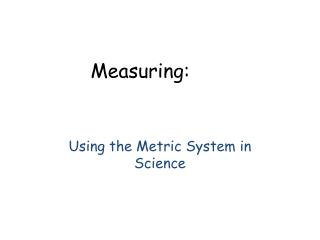DownloadDownload PresentationMeasuring:

# Measuring:

Télécharger la présentation## Measuring:

- - - - - - - - - - - - - - - - - - - - - - - - - - - E N D - - - - - - - - - - - - - - - - - - - - - - - - - - -
##### Presentation Transcript

1. Measuring: Using the Metric System in Science

3. A graduated cylinder is used to measure liquid volume. It is the most accurate of the glassware used to measure liquid volume. The milliliter is the unit of measure for liquid volume.

4. metric ruler

5. A metric ruler is used to measure length or distance.The unit of measure is a centimeter (cm) or millimeter (mm).

6. beaker

7. A beaker is glassware that is used to measure liquid volume.The unit of measure is the milliliter.

8. triple beam balance

10. A flask is glassware that is used to measure liquid volume.The unit of measure is the milliliter (ml).

11. Match the following measuring tools to their unit of measure and type of measurement.

12. Question 1A graduated cylinder measures a) massb) liquid volumec) distancein what unit?d) millilitere) centimeterf) gram

13. Question 2A flask measuresa) liquid volumeb) massc) distancein what unit?d) millimetere) gramf) milliliter

14. Question 3A beaker measuresa) massb) liquid volumec) distancein what unit?d) millilitere) gramf) centimeter

15. Question 4Which is more accurate? • a beaker • a flask • a graduated cylinder

16. Question 5A triple beam balance measuresa) massb) liquid volumec) distancein what unit?d) millilitere) gramf) millimeter